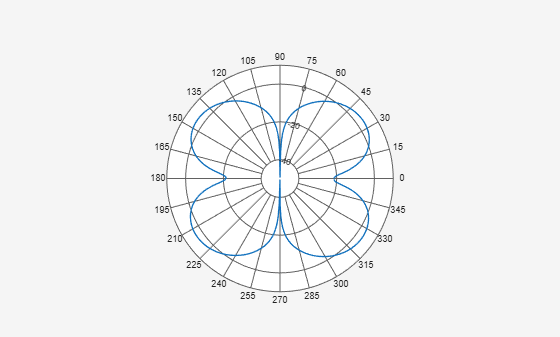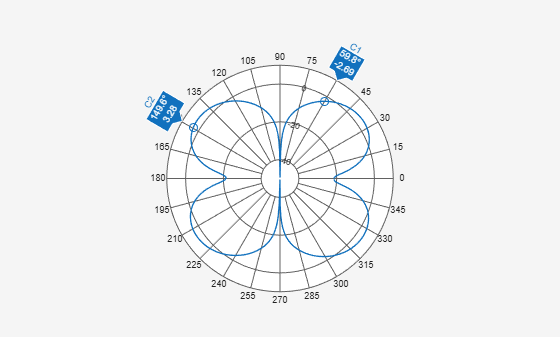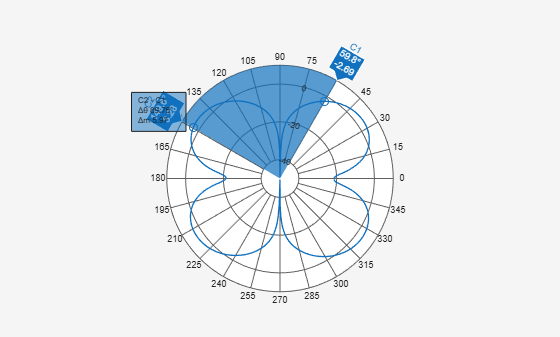# showSpan

Class: polarpattern

Show or hide angle span between two markers

## Syntax

``showSpan(p,id1,id2)``
``showSpan(p,id1,id2,true)``
``showSpan(p,vis)``
``showSpan(p)``
``d = showSpan(___)``

## Description

example

````showSpan(p,id1,id2)` displays the angle span between two angle markers, `id1` and `id2`. The angle span is calculated counterclockwise.```
````showSpan(p,id1,id2,true)` automatically reorders the angle markers such that the initial angle span is less than or equal to 180° counterclockwise. ```
````showSpan(p,vis)` sets angle span visibility by setting `vis` to `true` or `false`.```
````showSpan(p)` toggles the angle span display on and off. ```
````d = showSpan(___)` returns angle span details in a structure, `d` using any of the previous syntaxes.```

## Input Arguments

expand all

Polar plot, specified as a scalar handle.

Cursor or peak marker identifiers, specified as character vector. Adding cursors to the polar plot creates cursor marker identifiers. Adding peaks to the polar plot creates peak marker identifiers.

Example: `showspan(p,'C1','C2')`. Displays the angle span between cursors, `C1` and `C2` in polar plot, `p`.

## Show Angle Span

Create a dipole antenna and plot the directivity at 270 MHz.

```d = dipole; D = pattern(d,270e6,0,0:1:360); p = polarpattern(D);```Add cursors to the polar plot at approximately 60 and 150 degrees.

`addCursor(p,[60 150]);`Show the angle span between the two angles.

`showSpan(p,'C1','C2');`## Version History

Introduced in R2016a